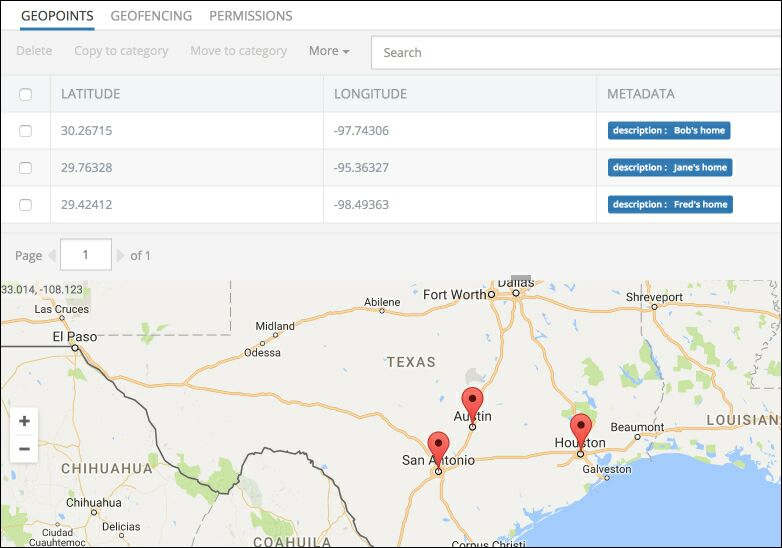# Search by distance¶

With the ability to store spatial data in the database,you can search for data objects by distance. This type of search returns a paged set of data objects which satisfy the `whereClause` condition and located within the specified distance. Distance-based search uses a special function in `whereClause` of the search request. The syntax of the function is:

``````distance(
center point latitude,
center point longitude,
columnname which contains geo point.latitude,
columnname which contains geo point.longitude )<operator> units-function(value)
``````

where:

Argument                Description
`<operator>` Possible values are <, >, =, >=, <=
`units-function` Defines the units of measure for the distance. Possible values are:
`ft( X )` - the distance value X is expressed in feet
`km( X )` - the distance value X is expressed in kilometers
`mi( X )` - the distance value X is expressed in miles
`yd( X )` -  the distance value X is expressed in yards

For example, the following `whereClause` expression searches for data objects located within 200 miles from the point at `30.26715, -97.74306`. Each data object must have the `coordinates` property of type GeoPoint.

``````distance( 30.26715, -97.74306, coordinates.latitude, coordinates.longitude ) < mi(200)
``````

The following example demonstrates a search-by-distance query. The example uses three data objects stored in the `Friend` table: Bob, Jane, and Fred who respectively live in Austin, Houston, San Antonio. The search query in the example finds all friends who live within the specified distance. Before running the search query, create the objects in the data storage with the corresponding geo points.

The application must be setup with demo data in order to run the example and see the `distance` operator in action. See the setup code in the expandable block below:

Setting up the app with demo data.

The`Friend` class definition:

You do not need to declare/write any custom classes when using the Dictionary approach. Database objects are represented as `Dictionary<string, object>` objects. Column names become dictionary key names and the object values are the corresponding values. === "Custom Class"

The Friend class definition:

``````class Friend
{
public string Name { get; set; }
public String PhoneNumber { get; set; }
public GeoPoint Coordinates { get; set; }
}
``````

Run the following query/code to store a data object representing Bob with a link to his home in Austin, TX:

``````Dictionary<string, object> bob = new Dictionary<string, object>();
bob[ "name" ] = "Bob";
bob[ "phoneNumber" ] = "512-555-1212";
bob = Backendless.Data.Of( "Friend" ).Save( bob );

GeoPoint geopoint = new GeoPoint( 29.76328, -95.36327 );
geopoint = Backendless.Geo.SavePoint( geopoint );

Backendless.Data.Of( "Friend" ).SetRelation( bob, "coordinates:GeoPoint:1", new object[] { geopoint } );
``````
``````Friend bob = new Friend();
bob.Name = "Bob" ;
bob.PhoneNumber = "512-555-1212";
bob = Backendless.Data.Of<Friend>().Save( bob );

GeoPoint geopoint = new GeoPoint( 29.76328, -95.36327 ) );
geopoint = Backendless.Geo.SavePoint( geopoint );

Backendless.Data.Of<Friend>().SetRelation( bob, "Coordinates:GeoPoint:1", new object[]{geopoint} );
``````

Run the following query/code to store a data object representing Jane with a link to her home in Houston, TX:

``````Dictionary<string, object> jane = new Dictionary<string, object>();
jane[ "name" ] = "Jane";
jane[ "phoneNumber" ] = "281-555-1212";
jane = Backendless.Data.Of( "Friend" ).Save( jane );

GeoPoint geopoint = new GeoPoint( 29.76328, -95.36327 );
geopoint = Backendless.Geo.SavePoint( geopoint );

Backendless.Data.Of( "Friend" ).SetRelation( jane, "coordinates:GeoPoint:1", new object[] { geopoint } );
``````
``````Friend jane = new Friend();
jane.Name = "Jane" ;
jane.PhoneNumber = "281-555-1212";
jane = Backendless.Data.Of<Friend>().Save( jane );

GeoPoint geopoint = new GeoPoint( 29.76328, -95.36327 ) );
geopoint = Backendless.Geo.SavePoint( geopoint );

Backendless.Data.Of<Friend>().SetRelation( jane, "Coordinates:GeoPoint:1", new object[]{geopoint} );
``````

Run the following query/code to store a data object representing Fred with a link to his home in San Antonio, TX:

``````Dictionary<string, object> fred = new Dictionary<string, object>();
fred[ "name" ] = "Fred";
fred[ "phoneNumber" ] = "210-555-1212";
fred = Backendless.Data.Of( "Friend" ).Save( fred );

GeoPoint geopoint = new GeoPoint( 29.42412, -98.49363 );
geopoint = Backendless.Geo.SavePoint( geopoint );

Backendless.Data.Of( "Friend" ).SetRelation( fred, "coordinates:GeoPoint:1", new object[] { geopoint } );
``````
``````Friend fred = new Friend();
fred.Name = "Fred" ;
fred.PhoneNumber = "210-555-1212";
fred = Backendless.Data.Of<Friend>().Save( fred );

GeoPoint geopoint = new GeoPoint( 29.42412, -98.49363 ) );
geopoint = Backendless.Geo.SavePoint( geopoint );

Backendless.Data.Of<Friend>().SetRelation( fred, "Coordinates:GeoPoint:1", new object[]{geopoint} );
``````

Once the data is in the database and the geo location storage, you can verify it in Backendless Console by opening the Geolocation screen and selecting the Home geocategory:Suppose you need to get all objects located within 300 miles radius from `Beaumont, TX`, which has the GPS coordinates of `30.084, -94.145`. The following code/query performs that distance-based search:

``````String whereClause = "distance( 30.26715, -97.74306, " +
"coordinates.latitude, coordinates.longitude ) < mi(200)";
DataQueryBuilder queryBuilder = DataQueryBuilder.Create();
queryBuilder.SetWhereClause( whereClause ).SetRelationsDepth( 1 );
IList<Dictionary<string, object>> friends = Backendless.Data.Of( "Friend" ).Find( queryBuilder );

String format = "{0} lives at {1}, {2} tagged as '{3}'";

foreach( Dictionary<string, object> friend in friends )
{
GeoPoint coordinates = (GeoPoint) friend[ "coordinates" ];
System.Console.WriteLine( String.Format( format,
friend[ "name" ],
coordinates.Latitude,
coordinates.Longitude,
(String) coordinates.Metadata[ "description" ] ) );
}
``````
``````String whereClause = "distance( 30.26715, -97.74306, " +
"coordinates.latitude, coordinates.longitude ) < mi(200)";
DataQueryBuilder queryBuilder = DataQueryBuilder.Create();
queryBuilder.SetWhereClause( whereClause ).SetRelationsDepth( 1 );
IList<Friend> friends = Backendless.Data.Of<Friend>().Find( queryBuilder );

String format = "{0} lives at {1}, {2} tagged as '{3}'";

foreach( Friend friend in friends )
{
GeoPoint coordinates = friend.Coordinates;
System.Console.WriteLine( String.Format( format,
friend.Name,
coordinates.Latitude,
coordinates.Longitude,
(String) coordinates.Metadata[ "description" ] ) );
}
``````

The search returns all data objects within the specified distance. Each data object has the `Coordinates` property containing the coordinates of a geo point associated with this data object.